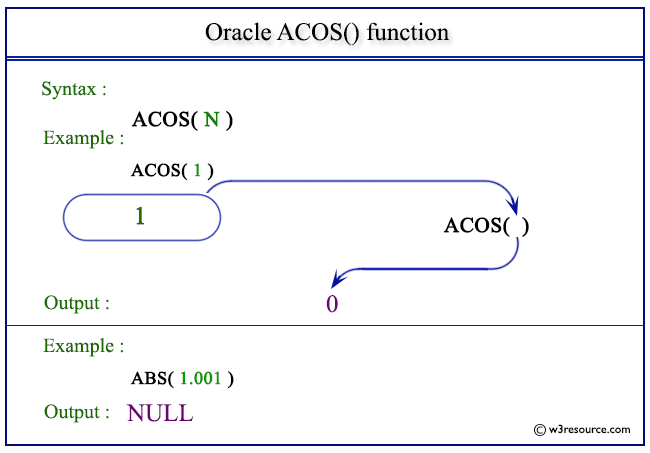# Oracle ACOS() function

## Description

The ACOS() function is used to calculate the angle value (in radians) of a specified cosine. The function returns a value in the range of 0 to pi, expressed in radians and returns an error message when the value of the number is not in the range -1 to 1.
The function takes any numeric or nonnumeric data type (can be implicitly converted to a numeric data type) as an argument.
If the argument is BINARY_FLOAT, then the function returns BINARY_DOUBLE. Otherwise, the function returns the same numeric data type as the argument.

Syntax:

`ACOS(N)`

Parameters:

Name Description
N A number whose arc cosine value is to be retrieved.

Pictorial Presentation of ACOS() functionExample

The following statement shows that the statement will return the arc cosine value of the number defined as an argument.

``````SELECT ACOS(1) FROM dual;
```
```

Here is the result.

```   ACOS(1)
----------
0
```

Example: ACOS() function using fractional number

The following statement will return arc cosine of the fractional value defined in an argument.

``````SELECT ACOS(.053211) FROM dual;
```
```

Here is the result.

```ACOS(.053211)
-------------
1.51756018
```

Example : ACOS() function using zero(0)

The statement below will return the arc cosine value of the number defined as an argument.

``````SELECT ACOS(0) FROM dual;
```
```

Here is the result.

```   ACOS(0)
----------
1.57079633
```

Previous: ABS
Next: ASIN

﻿